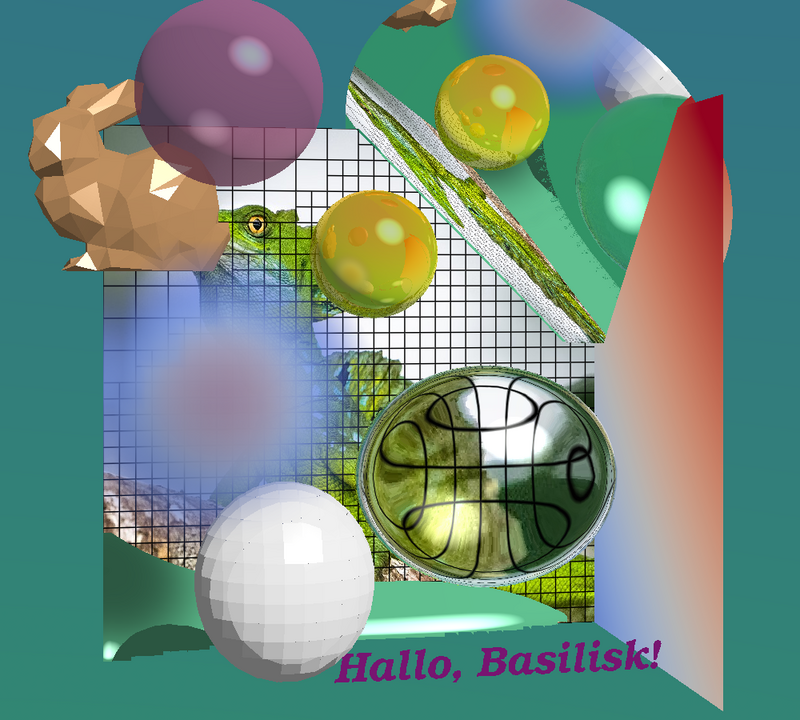# All bwatch sketch functionsA minimal example

From top left to bottom right, you see:

1. A background with a color gradient
2. A Stanford bunny, triangular tessellation by Johnny6 via Thingiverse
3. A semi transparent sphere
4. A slice of the grid
5. A photo by Kitty Kono via Flickr.
6. A volumetric smoke plume with contrentration color coding that is casting a shade on itself and the isosurface
7. A round mirror
8. A reflective sphere, color coded with scalar data
9. A green iso surface of scalar data
10. white fov facets that cast a shade
11. A refractive blob definded by volume fractions
12. A string
13. A slice displaying scalar field data
#include "grid/octree.h"
#include "bwatch.h"
#include "distance.h"

scalar dummy[], s[], f[], f2[], s2[];

void flipflop (coord * p) {
while (p->x < nodata) {
//resize
foreach_dimension()
p->x /= 300;
//rotate;
double tmp = p->y; p->y = p->z; p->z = tmp;
//mirror;
p->z *= -1;
//positioning
p->x -= 0.55;
p->y += 0.20;
p++;
}
}

int main() {
X0 = Y0 = Z0 = -L0/2.01;
init_grid (32);
unrefine (y - x > 0.45);
system ("wget -q \
https://cdn.thingiverse.com/assets/87/d8/89/b0/7c/Bunny-LowPoly.stl");
system ("wget -q \
https://live.staticflickr.com/7894/45505956245_f24c178bed_b.jpg");
coord * p = input_stl (fopen ("Bunny-LowPoly.stl", "r"));
flipflop (p);
foreach() {
s[] = cube(x) + y + z;
s2[] = 1.1*(exp(-(sq(x + 0.3) + sq(y) + sq(z - 0.25))*40.) - 0.05);
}
boundary ({s, s2});
fraction (f , (0.2  - sqrt((sq(x/1.2 - 0.15) + sq(y + 0.15) + sq(z - 0.15)))));
fraction (f2, (0.15 - sqrt((sq(x + 0.2) + sq((y + 0.3) /1.1) + sq(z - 0.3)))));
restriction ({f, f2});
watch (fov = 1.5, O = {-0.5, 0.2, 0}, poi = {0.05, 0.05, 0}, nx = 1000, ny = 900);
image ("45505956245_f24c178bed_b.jpg",
alpha = -0.2, res = 650, mat = {dull = true});
sphere (5, mat = {dull = true});
equiplane (f, vof = true, mat = {ind = 1.2});
sketch_vof (f2, true);
equiplane (s, -0.6);
volume (s2, cols = true, sc = 0.04, mval = 0.01, shading = 1);
quadriangles (s, n = {1,0,0}, alpha = 0.49, mat = {map = cool_warm, linear = true});
lattice (alpha = -0.2, HQ = true);
disk (0.4, {0.3, 0.4, 0}, {-1., -1., 1.});
sphere (C = {-0.0, 0.25, .0}, R = 0.12, mat = {s = s, linear = true, R = 0.3});
sphere (R = 0.15, C = {-0.3, 0.5, 0.4}, mat = {T = 0.5});
triangles (p);
sketch_text ("Hallo, Basilisk!  ", ops = "-font Bookman-DemiItalic",
pos = "southeast", tc = {131, 23, 123}, fs = 70, alpha = 0.3);

store (fopen ("min.ppm", "w"));
plain();
system ("convert min.ppm -resize 80% min.png"); //some MSAA
free (p);
}